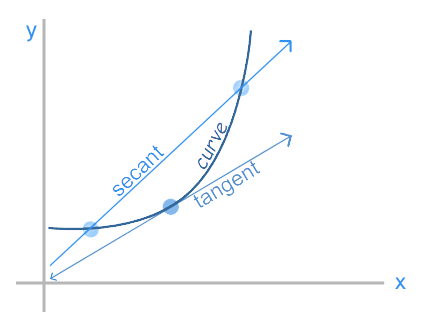# Tangent Line Calculator

## Find tangent lines step by step

The calculator will find the tangent line to the explicit, polar, parametric and implicit curve at the given point, with steps shown.

It can handle horizontal and vertical tangent lines as well.

The tangent line is perpendicular to the normal line.

Related calculator: Normal Line Calculator

If the calculator did not compute something or you have identified an error, or you have a suggestion/feedback, please write it in the comments below.

Our Tangent Line Calculator was created to help you find tangent lines. Whether you're a student or a researcher, our calculator will help you find the equation of a tangent line to an explicit, parametric, polar, or implicit curve.

## How to Use the Tangent Line Calculator?

• ### Input

Enter the function the specified input field. Use proper mathematical notation and symbols to represent the function accurately. Indicate the x-value at which you want to determine the tangent line. This point is where the tangent line will touch the function's curve. You can also choose the function type: explicit, parametric, polar, or implicit.

• ### Calculation

Click the "Calculate" button to process the function and display the result.

• ### Result

The calculator will output the equation of the tangent line at the specified point.

## What is Tangent Line?A tangent line is a fundamental concept that plays an important role in understanding the behavior of functions. This straight line has a special property: it touches a curve at a certain point (the point of tangency), having at this point the same slope and behavior as the function.

A tangent line to a function is a straight line that touches the function's curve at a single point, known as the point of tangency. Unlike a secant line, which passes through two points on the curve, a tangent line touches the curve at just one point.

The tangent line at a particular point on a curve shows the curve's instantaneous rate of change at that point. In other words, the slope of the tangent line is equal to the curve's slope at that point. As a result, the tangent line is a close approximation of the curve's behavior near the point of tangency.

Equation of a Tangent Line

The equation of the tangent line at the point $\left(x_0,f\left(x_0\right)\right)$ can be written using the point-slope form:

$$y-f\left(x_0\right)=f^{\prime}\left(x_0\right)\left(x-x_0\right),$$

where:

• $x_0$ is the x-coordinate of the point of tangency.
• $f\left(x_0\right)$ is the y-coordinate of the point of tangency.
• $f^{\prime}\left(x_0\right)$ denotes the derivative of the function $f(x)$ evaluated at $x=x_0$, i.e. the slope of the tangent line.
• $x$ is the x-coordinate of a point on the tangent line.
• $y$ represents the y-coordinate of a point on the tangent line.

Example

Consider the function $f(x)=x^2$ and the point $x=2$.

The slope of the tangent line at $x=2$ can be found by evaluating the derivative:

$$f^{\prime}(x)=2x$f^{\prime}(2)=2\cdot2=4 The equation of the tangent line can be written as follows: y-f(2)=4(x-2)$y-2^2=4(x-2)$$

Simplifying, we get that the equation of the tangent line to $f(x)=x^2$ at $x=2$ is $y=4x-4$.

## Why Choose Our Tangent Line Calculator?

• ### Accuracy

Our calculator provides accurate calculations of tangent lines, ensuring correct results for your mathematical problems and researches.

• ### User-Friendly Interface

Our calculator is easy to use and easy to navigate.

• ### Step-by-Step Solutions

The calculator helps you understand tangent lines by providing step-by-step solutions.

• ### Developed by Experts

Our calculator is built by math and educational technology experts to ensure accuracy and correctness.

### FAQ

#### What is tangent line?

A tangent line is a straight line that touches a curve at a single point (called the point of tangency), matching the curve's slope at that point.

#### Is the equation of the tangent line accurate?

The calculator uses the derivative to calculate the slope, ensuring correct equations of tangent lines.

#### Does the calculator give step by step solutions?

Yes, the calculator offers step-by-step solutions to better understand the concept of tangent lines or just to check your work.

#### Is the calculator an educational tool?

Yes, our calculator serves as an educational resource to help both students and educators deepen their understanding of tangent lines.